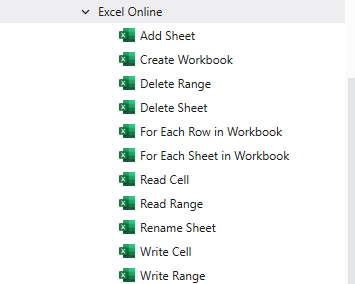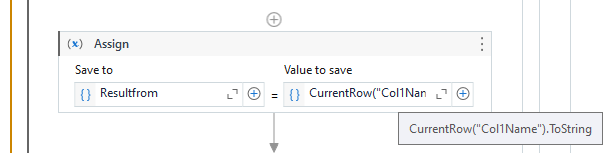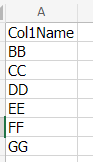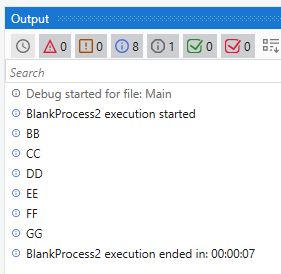# Read each cell in row in excel online one drive

i want to use read cell activities in each row in excel online, but it don’t let me to open the workbook bar because i am already in each row. How can i read each row? in the picture below you can see that i want to read to column from sheet" Number and names "

You can install Microsoft 365 package to use below activitiesAs the workbook is not local, you have to use the above activities

Thanks,
Srini

Can you try below approach-

If you want to read each row in an Excel online workbook using UiPath and you are unable to access the workbook bar because you are already within a row, you can use the following steps:

1. Use the “Excel Application Scope” activity in UiPath to open the Excel online workbook.
2. Use the “Read Range” activity inside the “Excel Application Scope” activity to read the data from the “Number and names” sheet in the workbook into a DataTable variable.
3. Use a “For Each Row” activity to iterate through each row in the DataTable variable.
4. Inside the “For Each Row” loop, you can use the “Read Cell” activity to read the data from each cell in the current row.
5. To specify the cell you want to read, you can use the row index and column name or column index as inputs to the “Read Cell” activity. For example, if you want to read data from column A and column B in each row, you can use “A” and “B” as the column names or “0” and “1” as the column indices.
6. You can store the read data in variables or process it as needed within the loop.

Thanks!

@sirini84 I am using Microsoft 365, as you can see in the picture i cant use the workbook

Hello @Nitya1
i am using excel online not normal excel, I don’t not work like this :s

Maybe you can try as below

Thanks,
Srini

Try use read range instead and save result into DT variable then use for each row in datatable to get cell value like picture below
Workflow :Data :Output :Best Regard,

Thank you @Srini84, but still I am stuck in how can I read each row in the data table. let’s assume that I want only the cell value in column A and Column B. How can i do it ?

Once you have the data in Datatable there is no concept of ColumnA and Column B, but there will be the Headers let suppose In Column A header name is Name and ColumnB header name is Age

Now using For Each row in Datatable activity you can pass the datatable which you get from Read Range activity and now to get the Name header value

You can write as CurrentRow(“Name”).ToString like wise for getting the Age you can write as CurrentRow(“Age”).ToString

Thanks,
Srini

Thank you so much, i will try it

Did you pass the Datatable variable correctly?
Because this Object reference error usually you get if there is a null values to the variable

Check that Read Range activity is working fine and having rows and columns

Also you can put a breakpoint and check in Locals whether the Datatable variable is reading correctly

Thanks,
Srini

and change row(“Colname1”).toString into row(“yourcolumnname”).toString
if you want to extract more column you can create one more variable like this
using assign activity
To : ResultFromCol1 Value : row(“Number”).toString
To : ResultFromCol2 Value : row(“Name”).toString